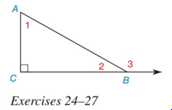Chapter 2.4, Problem 26E### Elementary Geometry for College St...

6th Edition
Daniel C. Alexander + 1 other
ISBN: 9781285195698

#### Solutions

Chapter
Section### Elementary Geometry for College St...

6th Edition
Daniel C. Alexander + 1 other
ISBN: 9781285195698
Textbook Problem
104 views

#In Exercises 25 to 27 , see the figure for exercise 24 . Given: m ∠ 1 = x , m ∠ 2 = x 2 Find: x

To determine

To find:

The value of x.

Explanation

Given:

m1=x,

And,

m2=x2

Figure (1)

Calculation:

Sum of acute angles in a right triangle is equal to 90°.

Equate the sum of acute angles in a right triangle to 90°.

m1+m2=90°(1)

Substitute x for m

### Still sussing out bartleby?

Check out a sample textbook solution.

See a sample solution

#### The Solution to Your Study Problems

Bartleby provides explanations to thousands of textbook problems written by our experts, many with advanced degrees!

Get Started

#### Simplify: 330360

Elementary Technical Mathematics

#### In Exercises 1124, find the indicated limits, if they exist. 15. limx2x+3x29

Applied Calculus for the Managerial, Life, and Social Sciences: A Brief Approach

#### Calculate y'. 7. y=t41t4+1

Single Variable Calculus: Early Transcendentals, Volume I

#### The area of the shaded region is given by:

Study Guide for Stewart's Single Variable Calculus: Early Transcendentals, 8th